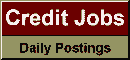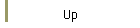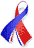DefaultRisk.com the web's biggest credit risk modeling resource.doi> search: A or B Export citation to:- HTML- Text (plain)- BibTeX- RIS- ReDIF

Compound Scenarios: An efficient framework for integrated market-credit risk

by Ben De Prisco of Algorithmics, Inc.,
Ian Iscoe of Algorithmics, Inc.,
Yijun Jiang of Algorithmics, Inc., and
Helmut Mausser of Algorithmics, Inc.

May 9, 2007

Abstract: This paper describes an efficient three-tiered scenario generation framework for assessing joint market-credit risk, with Monte Carlo simulation. The framework employs a set of so-called compound scenarios, having a tree-like structure comprising three levels, or tiers. The scenarios underlie correlated market and systemic credit factors and independent, idiosyncratic, credit-risk factors. We obtain confidence intervals for the mean and quantiles of a portfolio loss distribution in the non-iid setting of compound scenarios. The confidence intervals derive from the asymptotic normality of the sample mean and sample quantile and a variance decomposition formula that expresses the asymptotic variance in terms of the number of samples from each of the three tiers. The resultant formula directly measures the impact of various sample sizes on the variance of the estimate, which is more efficient than the trial-and-error approach used by practitioners today. Moreover, the formula allows one to optimise the choice of sample sizes, to minimise the estimator's variance subject to constraints on available time or computer resources. The results are illustrated with some numerical and empirical examples.

Keywords: integrated market credit risk, scenario generation, optimal sample size, sampling error, value at risk, confidence interval.

Books Referenced in this paper:  (what is this?)